Next: 2.3.1 Thermal Simulation Up: 2. Circuit Equations Previous: 2.2 Modified Nodal Approach

# 2.3 Constitutive Relation Formulation

To be compatible with the constitutive relation formulation used for the transport equations ( f(x) = 0) the constitutive relations for the device compact models are formulated in the same way rather than directly calculating linearized circuit elements. MINIMOS-NT solves for f(x) = 0 using a Newton algorithm:
 -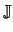k . u = f(xk) (2.18) xk + 1 = xk + u (2.19)k =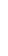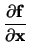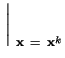. (2.20)

This formulation has the advantage that the error in the sum of branch currents is available as the residuum of the equations system at each iteration. Furthermore, the implementation of non-linear constitutive relations is simpler than deriving a linearized companion model. The original MNA formulation can be related to the above formulation by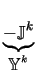.  xk + 1 =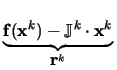. (2.21)

Equation (2.21) is of fundamental importance as it allows for conversion between models implemented for SPICE into MINIMOS-NT models by general purpose wrapper functions. Only the right hand side needs to be modified to transform the values obtained from SPICE model call into the stamps needed by MINIMOS-NT.

In MINIMOS-NT the constitutive relations for the node voltages are defined to be the sum of all currents flowing into this node which must be zero. There is no separation between passive elements and source terms. It is to note that the opposite direction of current flow originates in (2.18) where the negative derivatives are used to assemble the system matrix. This definition is, of course, arbitrary, but defining the current direction this way positive main-diagonal elements are obtained as in the conventional formulation.

The constitutive relation for each node voltage is the sum of the device currents flowing into the node.

 f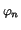=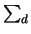fd=Idn = 0 (2.22)

Branch currents are normally introduced for voltage sources and ammeters. The latter is a voltage source with zero branch voltage which introduces the branch current into the system matrix so that it can be used to control another element. The branch current constitutive relations state relationships between node voltages, e.g.,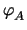-= V0.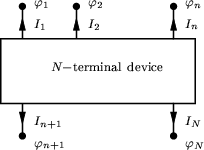For an N-terminal device with B branch currents, one gets N + B device relations.

 fd =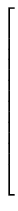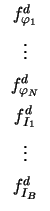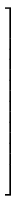=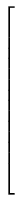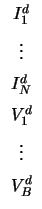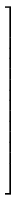with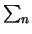Idn = 0 (2.23)

The partial contribution of the device d to the Jacobian matrix is given byd =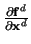(2.24)

where xd contains all unknowns which control the device behavior.Next: 2.3.1 Thermal Simulation Up: 2. Circuit Equations Previous: 2.2 Modified Nodal Approach
Tibor Grasser
1999-05-31